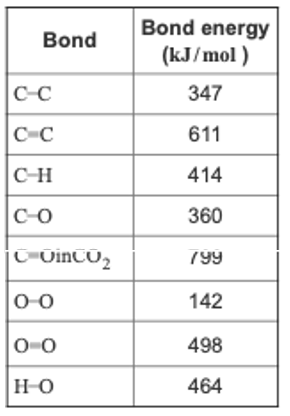# Problem: Calculate the bond energy per mole for breaking all the bonds in methane, CH4.Express your answer to four significant figures and include the appropriate units.In chemical reactions, heat is converted into chemical energy (the potential energy stored in chemical bonds) or vice versa. Bond energy is the energy required to break one mole of the bond in the gas phase. Since it takes energy to break a bond, bond energies are always positive. Conversely, energy is released when bonds are formed. Thus, the enthalpy change for a reaction can be approximated fromΔHrxn = ∑ (ΔH breaking) + ∑ (ΔH forming)where HH represents bond energies for the breaking (positive bond energy) or forming (negative bond energy) of a bond and HrxnH_rxn represents the overall enthalpy for the reaction.Use the table to answer questions about bond energies.

###### FREE Expert Solution
82% (477 ratings)View Complete Written Solution
###### Problem Details

Calculate the bond energy per mole for breaking all the bonds in methane, CH4.Express your answer to four significant figures and include the appropriate units.

In chemical reactions, heat is converted into chemical energy (the potential energy stored in chemical bonds) or vice versa. Bond energy is the energy required to break one mole of the bond in the gas phase. Since it takes energy to break a bond, bond energies are always positive. Conversely, energy is released when bonds are formed. Thus, the enthalpy change for a reaction can be approximated from

ΔHrxn = ∑ (ΔH breaking) + ∑ (ΔH forming)

where HH represents bond energies for the breaking (positive bond energy) or forming (negative bond energy) of a bond and HrxnH_rxn represents the overall enthalpy for the reaction.